# Algebra Seminars

## Seminars are held in 358 Parker Hall on Tuesdays at 2:30pm.

Upcoming Algebra Seminars
DMS Algebra Seminar
Oct 03, 2023 02:30 PM
358 Parker Hall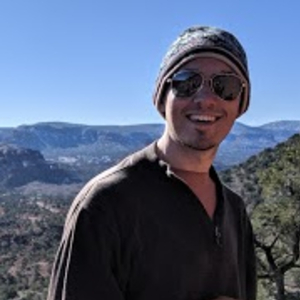Speaker: Keller VandeBogert (Notre Dame University)

Title: Stable Sheaf Cohomology via A-infinity Koszul Duality

Abstract: In arbitrary characteristic, it is known that the cohomology of line bundles on flag varieties eventually behaves like a fixed polynomial functor, depending only on the line bundle. Computing this "stable" polynomial functor remains a highly nontrivial open question with relatively few cases being known explicitly. In this talk, I will illustrate how the computation of stable cohomology may be significantly simplified by instead bundling sheaf theoretic data together as an algebra, then taking advantage of the A-infinity analogue of Koszul duality. This is joint work with Claudiu Raicu.

Past Algebra Seminars
DMS Algebra Seminar
Aug 29, 2023 02:30 PM
358 Parker Hall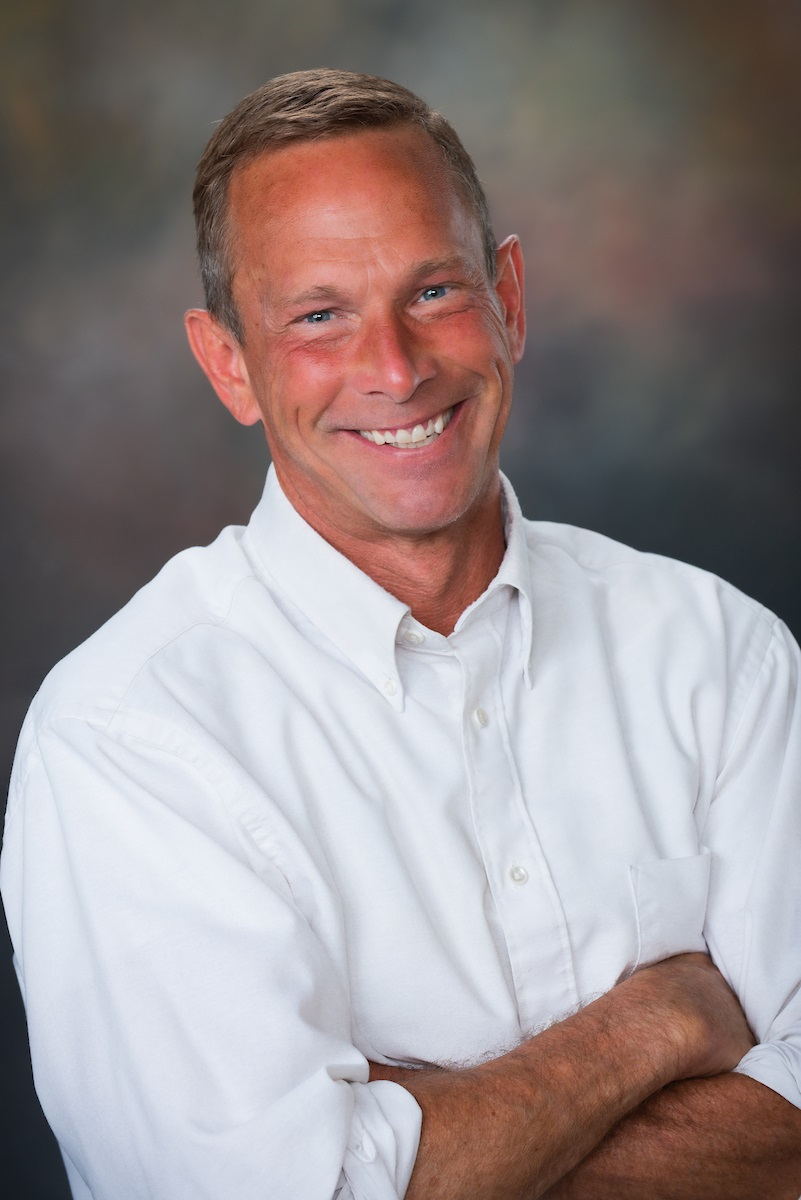Speaker: Hal Schenck, Auburn University

Title: Kuramoto Oscillators: Algebraic and Topological Aspects

Abstract: We investigate algebraic and topological signatures of networks of coupled oscillators. Translating dynamics into a system of algebraic equations enables us to identify classes of network topologies that exhibit unexpected behaviors. Many previous studies focus on synchronization of networks having high connectivity, or of a specific type (e.g., circulant networks). We introduce the Kuramoto ideal; an algebraic analysis of this ideal allows us to identify features beyond synchronization, such as positive dimensional components in the set of potential solutions (e.g., curves instead of points). We prove sufficient conditions on the network structure for such solutions to exist. The points lying on a positive dimensional component of the solution set can never correspond to a linearly stable state. We apply this framework to give a complete analysis of linear stability for all networks on at most eight vertices. Furthermore, we describe a construction of networks on an arbitrary number of vertices having linearly stable states that are not twisted stable states. Joint work with M. Stillman (Cornell) and H. Harrington (Oxford).

DMS Algebra Seminar
Aug 22, 2023 02:30 PM
358 Parker Hall

Speaker: Michael Brown (Auburn University)

Title: Orlov’s Landau-Ginsburg/Calabi-Yau Correspondence

Abstract: I will discuss a landmark theorem of Orlov that relates the derived category of sheaves on a projective complete intersection to the singularity category of its affine cone. I will also discuss work in progress with Prashanth Sridhar, where we aim to generalize Orlov's theorem to the setting of differential graded algebras.

DMS Algebra Seminar
Apr 18, 2023 02:30 PM
358 Parker HallSpeaker: Xianglong Ni (UC-Berkeley)

Title: Schubert varieties and the structure of codimension three perfect ideals

Abstract: In the orthogonal Grassmannian OG$$(n,2n)$$, there are two (opposite) Schubert varieties of codimension three. Their restrictions to the big affine open cell are cut out by equations familiar to commutative algebraists. For example, one is defined by the submaximal Pfaffians of a generic skew matrix---thus, by the structure theorem of Buchsbaum and Eisenbud, it is the universal example of a Gorenstein ideal of codimension three on $$n$$ generators. The other gives the universal example of an almost complete intersection of type $$n-3$$. I will explain how this example relates to Weyman's work on generic free resolutions of length three, and how it conjecturally extends to perfect ideals with other particular Betti numbers.

DMS Algebra Seminar
Apr 11, 2023 02:30 PM
358 Parker Hall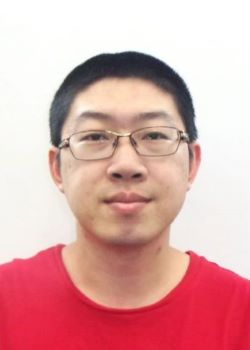Speaker: Cheng Meng (Purdue)

Title: Decompositions of local cohomology tables

Abstract: Let R be a standard graded polynomial ring over a field k, then the Boij-S\"oderberg theory describes the cone spanned by the Betti tables of finitely generated graded R-modules. This theory shows that the extremal rays of the cone are given by the pure Betti tables and every Betti table is a finite linear combination of pure Betti tables with positive rational coefficients. In this talk, we will focus on the cone spanned by the local cohomology tables of finitely generated graded R-modules of projective dimension at most 1. We will describe the extremal rays of the cone generated by local cohomology tables of such modules and show that not every local cohomology table of such a module is a finite linear combination of the tables on the extremal ray with positive rational coefficients.

DMS Algebra Seminar
Apr 04, 2023 02:30 PM
358 Parker Hall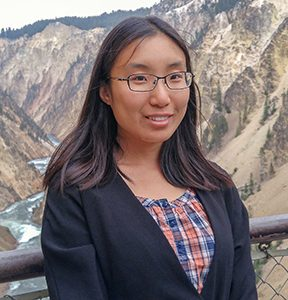Speaker: Wanlin Li (Washington University in St. Louis)

Title: Algebraic and arithmetic properties of curves via Galois cohomology

Abstract: A lot of the algebraic and arithmetic information of a curve is contained in its interaction with the Galois group. This draws inspiration from topology, where given a family of curves over a base B, the fundamental group of B acts on the cohomology of the fiber. As an arithmetic analogue, given an algebraic curve C defined over a non-algebraically closed field K, the absolute Galois group of K acts on the etale cohomology of the geometric fiber and this action gives rise to various Galois cohomology classes. In this talk, we discuss how to use these classes to detect algebraic and arithmetic properties of the curve, such as the rational points (following Grothendieck's section conjecture) and whether the curve is hyperelliptic.

DMS Algebra Seminar
Mar 28, 2023 02:30 PM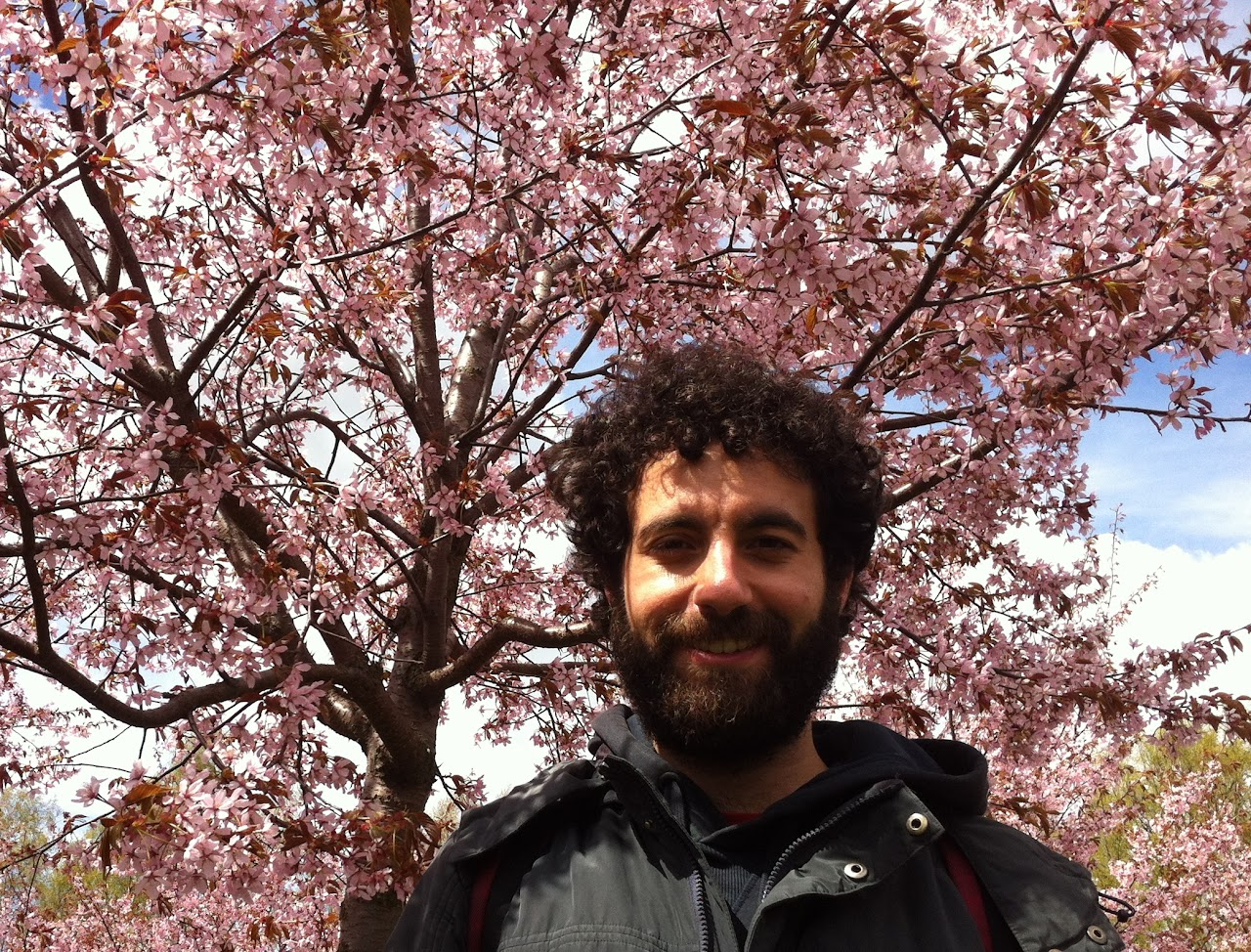Speaker: Emanuele Ventura, Politecnico di Torino (Italy)

Title: Implicitisation and parameterisation in polynomial functors

Abstract: Closed subsets of polynomial functors are highly symmetrical varieties that live in infinite dimensions. Due to these large symmetries, they can be defined by finitely many polynomial equations. This is a recent result of Draisma. In this talk, I will discuss how to make this and more recent results of Bik-Draisma-Eggermont-Snowden algorithmic. Our algorithms use classical elimination in finite but ever-increasing dimension, but also separate routines, with no classical analogue, which search for a certificate that one has found enough equations.

DMS Algebra Seminar
Mar 23, 2023 02:30 PM
358 Parker Hall

PLEASE NOTE SEMINAR IS ON THURSDAY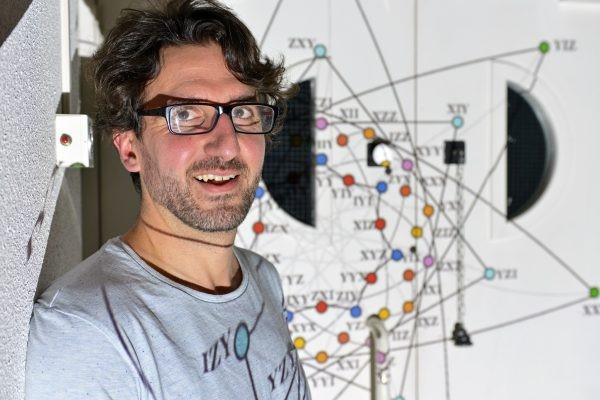Speaker: Fred Holweck, Université de Technologie de Belfort-Montbéliard

Title: Spin representation and Fermionic Fock space

Abstract: In Quantum information, it is standard to study the classification of pure n-qubit states under the SLOCC (Stochastic Local Operations with Classical Communication) group. Mathematically, this problem boils down to the study of orbits in $V=(\CC^2)^{\otimes n}$ under the semi-simple Lie group $G=SL_2(\CC)^{\times n}$. But what about other Lie groups G and their representations V ? In this talk, after introducing a geometric framework to study classification of $n$-qubits quantum states in the language of auxiliary varieties of the highest weight orbit of the SLOCC group, I'll explain how similar constructions could be used for bosonic and fermionic states. In particular, I will talk about the relation between the spin representation and quantum states described in Fermionic Fock space. This last identification was used by Luke Oeding and myself to provide a new invariant useful for quantum information.

DMS Algebra Seminar
Mar 21, 2023 02:30 PM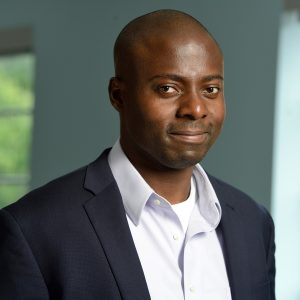Speaker: Edinah Gnang, Johns Hopkins University

Title: Bounds on the Chow-rank of hypermatrices via Partial Differential Encoding of Boolean functions

Abstract: We introduce partial differential encodings of Boolean functions and their relaxations. These encodings enable us to determine the fraction of optimal encodings of some important Boolean functions. Our main result is a general method for deriving upper and lower bounds on the Chow-Rank of hypermatrices which underlie polynomial transformed by some group or semi-group actions. Our method is a discrete analog of representation theory methods which devise bounds on the border rank via Lie group actions.

The talk is based on joint work with Rongyu Xu.

DMS Algebra Seminar
Feb 21, 2023 02:30 PM
358 Parker Hall

Speaker: Prashanth Sridhar, Auburn,

Title: Periodicity of Fitting Ideals in Free Resolutions

Abstract: We examine the periodicity of ideals of minors in minimal free resolutions of finitely generated modules over local rings with a focus on their asymptotic behavior. The heuristics rely on the general principle that the initial part of the resolution is influenced by the module’s relations, while the singularities of the ring have a telling effect on the asymptotic behavior. For example, we show that for any finite module over a local complete intersection, the Fitting ideals of any size in its minimal free resolution are eventually 2-periodic (although the resolution is not). Moreover, if the stable value of a given size of minors is non-zero, it contains some power of the Jacobian ideal. Results of this type hold true in other settings as well (ex: Golod rings or residue field over any local ring), but do not hold in general.

This is joint work with Michael K. Brown and Hailong Dao.

DMS Algebra Seminar
Feb 14, 2023 02:30 PM
358 Parker Hall

Speaker: Luke Oeding (Auburn)

Title: A hyperdeterminant on Fermionic Fock Space

Abstract: Twenty years ago Cayley's hyperdeterminant, the degree four invariant of a certain polynomial ring over the complex numbers under an action of a special linear group, was popularized in modern physics as it separates genuine entanglement classes in the three qubit Hilbert space and is connected to entropy formulas for special solutions of black holes. In this note we compute the analogous invariant on the fermionic Fock space for N=8, i.e., spin particles with four different locations, and show how this invariant projects to other well-known invariants in quantum information. We also give combinatorial interpretations of these formulas.